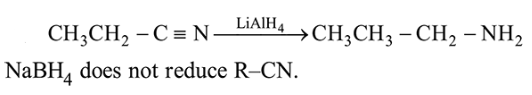# The most appropriate reagent for conversion ofQuestion:

The most appropriate reagent for conversion of $\mathrm{C}_{2} \mathrm{H}_{5} \mathrm{CN}$ into $\mathrm{CH}_{3} \mathrm{CH}_{2} \mathrm{CH}_{2} \mathrm{NH}_{2}$ is :

1. $\mathrm{NaBH}_{4}$

2. $\mathrm{CaH}_{2}$

3. $\mathrm{LiAlH}_{4}$

4. $\mathrm{Na}(\mathrm{CN}) \mathrm{BH}_{3}$

Correct Option: , 3

Solution: